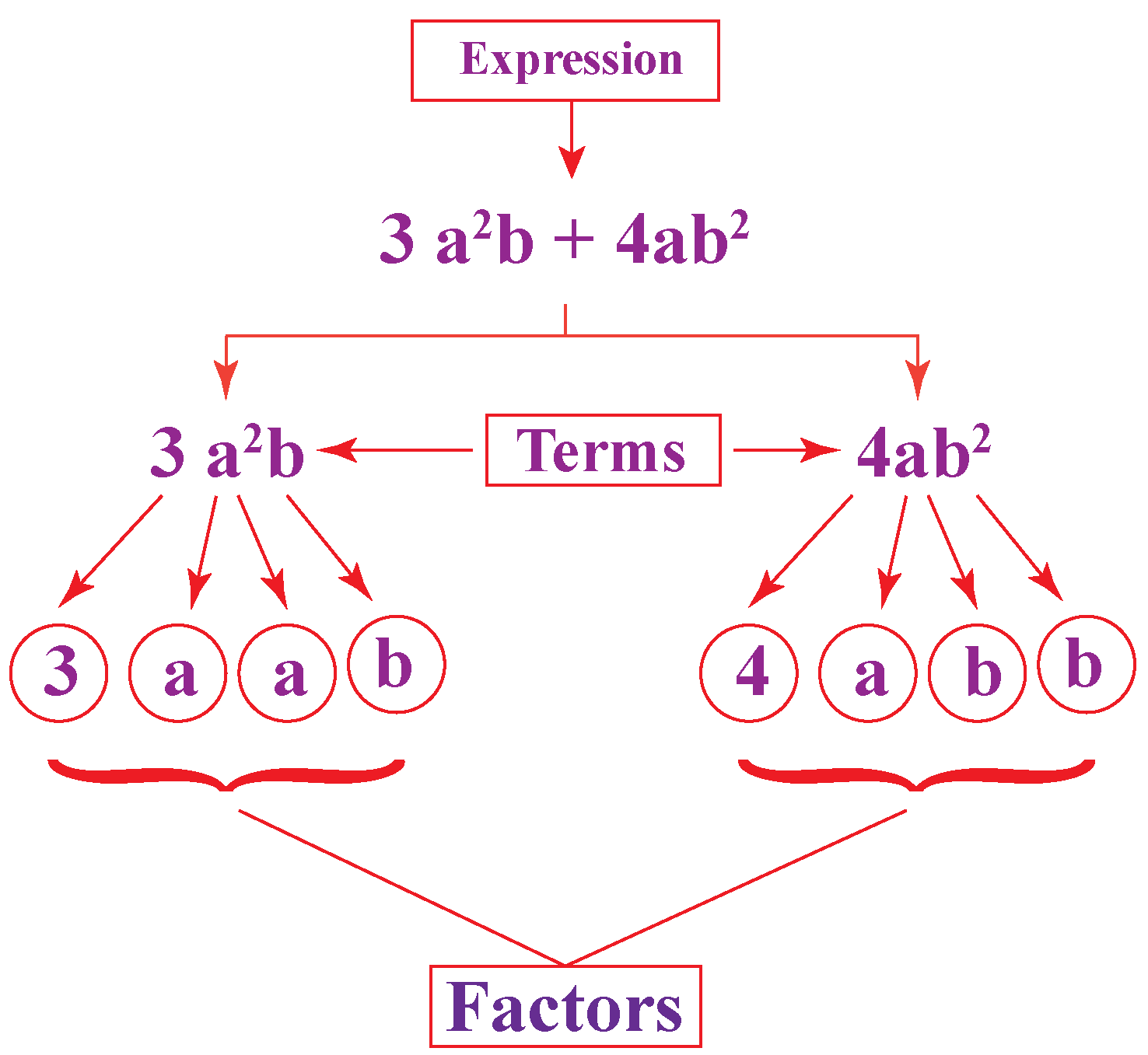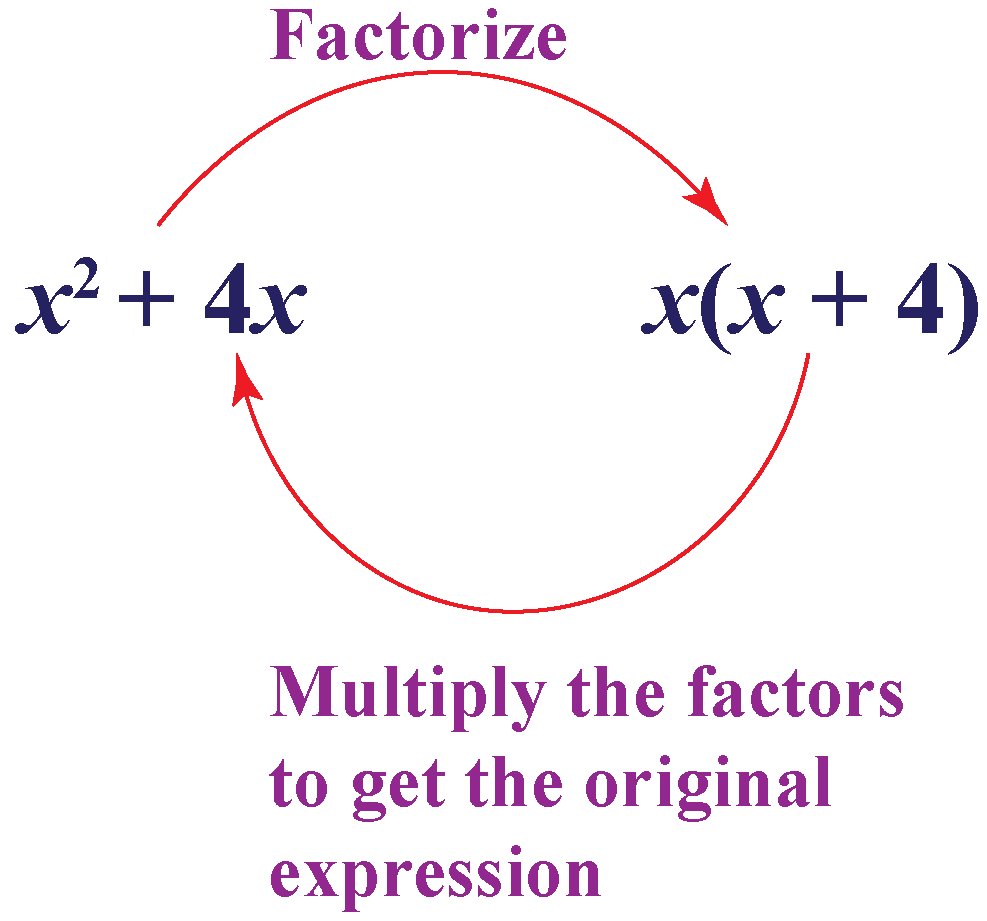# Factorization of Algebraic Expressions

Factorization of Algebraic Expressions

We all are familiar with the term, 'Factors'.

A factor is a number that divides the given number without any remainder. It simply means expressing a number as a multiplication of two other numbers. Similarly, in Algebra we write the algebraic expressions as a product of their factors. The only difference here is that an algebraic expression involves numbers and variables combined with an arithmetic operation like addition or subtraction.

In this lesson, we will learn about factorization, its meaning, how to factorize algebraic expressions using various methods, algebraic expressions and identities solved examples on factoring of polynomials and some interactive questions at the end.## Lesson Plan

 1 What Is Factorization of Algebraic Expressions? 2 Important Notes 3 Solved Examples 4 Tips and Tricks 5 Interactive Questions

## What Is Factorization of Algebraic Expressions?

'Factor' is a term used to express a number as a product of any two numbers. Factorization is a method of finding factors for any mathematical object, be it a number, a polynomial or any algebraic expression.

For example, the factors of 10 are 1,2,5 and 10. Similarly, an algebraic expression can also be factorized.

When the factors are multiplied they result in the original number or an expression that is factorized.

For example, consider the expression (x2+4x). It can be factorized as x(x+4)

When we multiply (x) and (x+4), we get the original expression (x2+4x)## How Do You Factorize Algebraic Expressions?

We know that an algebraic expression is made up of terms.

For example, the term \begin{align} 7xy \end{align} can be factorized as \begin{align} 7 \times x \times y \end{align}. This term cannot be factorized further.

Now let us see the methods used to factorize algebraic expressions.

### Highest Common Factor Method

\begin{align} 2x^2 + 4x\end{align}

Follow the steps given below to find the factors of the expression.

Step 1:

\begin{align} 2x^2 \end{align}  can be factorized as \begin{align} 2 \times x \times x \end{align}, and

\begin{align} 4x\end{align} can be factorized as \begin{align}2 \times 2 \times x \end{align}.

Step 2:

Find the greatest common factor of the two terms. Here, we see that \begin{align} 2x\end{align} is the greatest common factor. We keep this factor outside the brackets, divide the polynomial terms by this factor and write the remaining expression inside the brackets.

Step 3:

Thus, the expression is factorized as \begin{align} 2x(x + 2)\end{align}

### Applying Identities

This method involves formulas of algebraic expression for factorization.

### Example 1

\begin{align} x^2 + 6x + 9\end{align}

We see that there are no common factors for the three terms in the expression.

However, in the expression, we see that \begin{align} 9\end{align} is a perfect square.

In this case, we seek the help of algebraic identities to factorize the expression easily.

This expression looks similar to the identity: \begin{align}(a+b)^2 = a^2 + 2ab +b^2\end{align}

Comparing the given expression to the identity, we get

\begin{align}a = x, b = 3\end{align}

Therefore, the factors are \begin{align}(x+3)^2\end{align} or \begin{align}(x+3) (x+3)\end{align}

### Example 2

\begin{align}16x^2 - 25y^2\end{align}

In this expression, we see that there are no common factors.

Also, we see that this expression is of the form: \begin{align}a^2 - b^2 = (a+b)(a-b)\end{align}

Comparing the given expression to the identity, we get

\begin{align}a = 4x, b = 5y\end{align}

Therefore, the factors are \begin{align}(4x + 5y) (4x - 5y)\end{align}

## List of Identities to Factorize Algebraic Expressions

Listed below are the formulas of algebraic expressions.

• \begin{align}(a+b)^2 = a^2 + 2ab +b^2\end{align}
• \begin{align}(a-b)^2 = a^2 - 2ab +b^2\end{align}
• \begin{align}a^2 - b^2 = (a+b)(a-b)\end{align}
• \begin{align}a^3 + b^3 = (a+b)(a^2-ab+b^2)\end{align}
• \begin{align}a^3 - b^3 = (a-b)(a^2+ab+b^2)\end{align}
• \begin{align}(a+b)^3 = a^3 + 3a^2b+3ab^2+b^3\end{align}
• \begin{align}(a-b)^3 = a^3 - 3a^2b+3ab^2-b^3\end{align}
More Important Topics
Numbers
Algebra
Geometry
Measurement
Money
Data
Trigonometry
Calculus